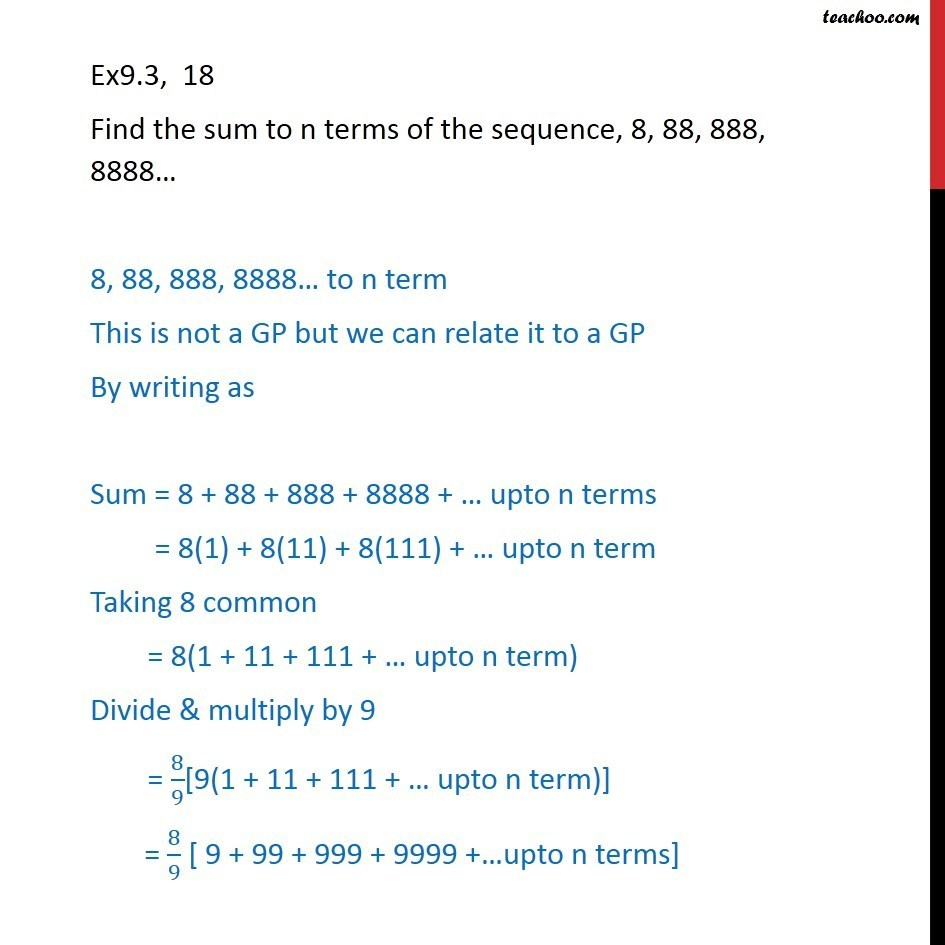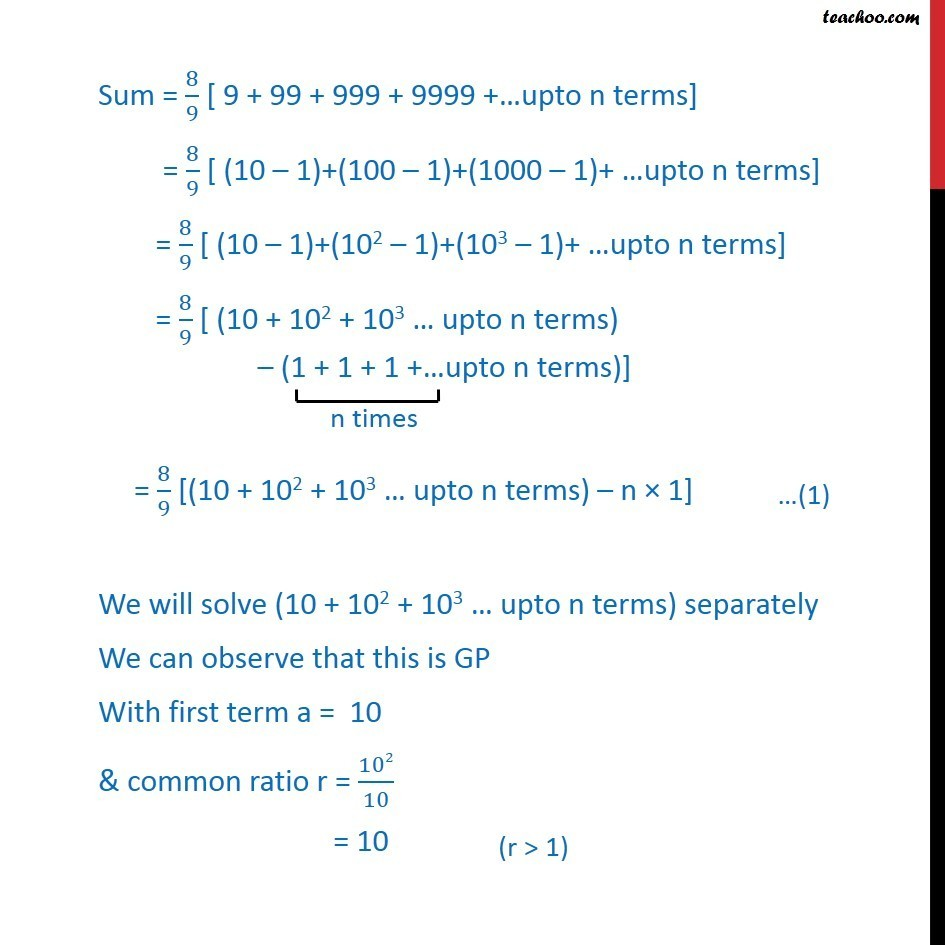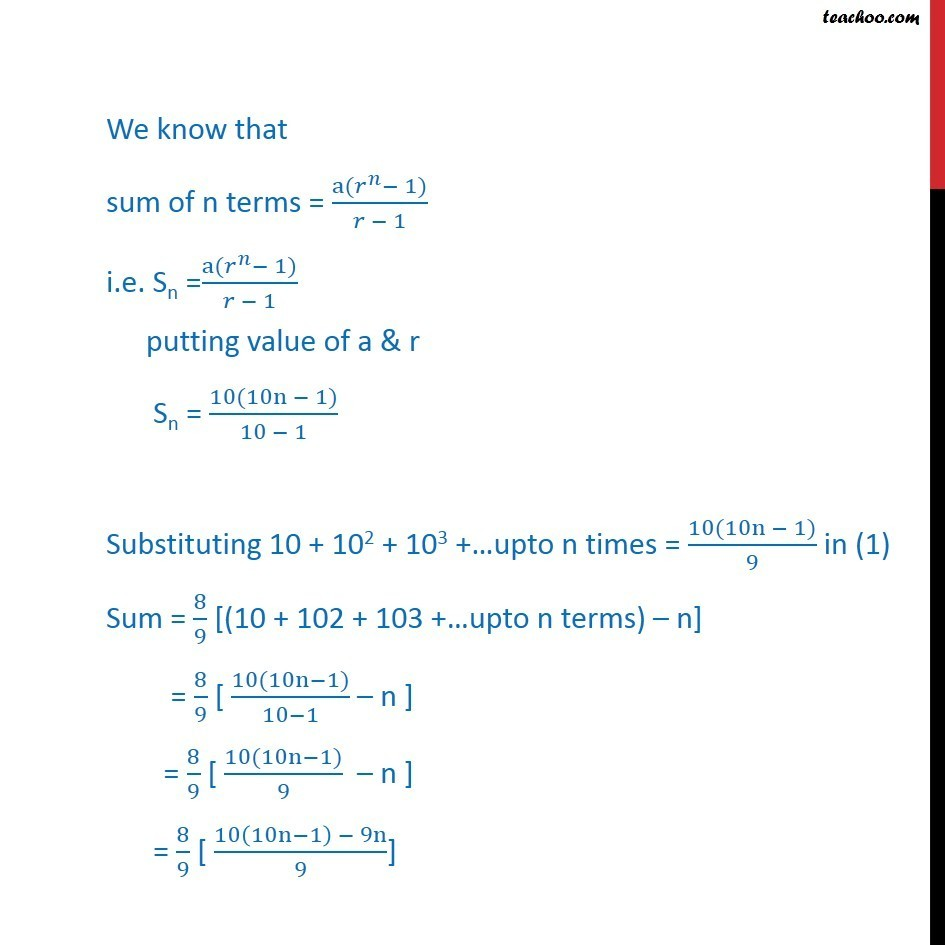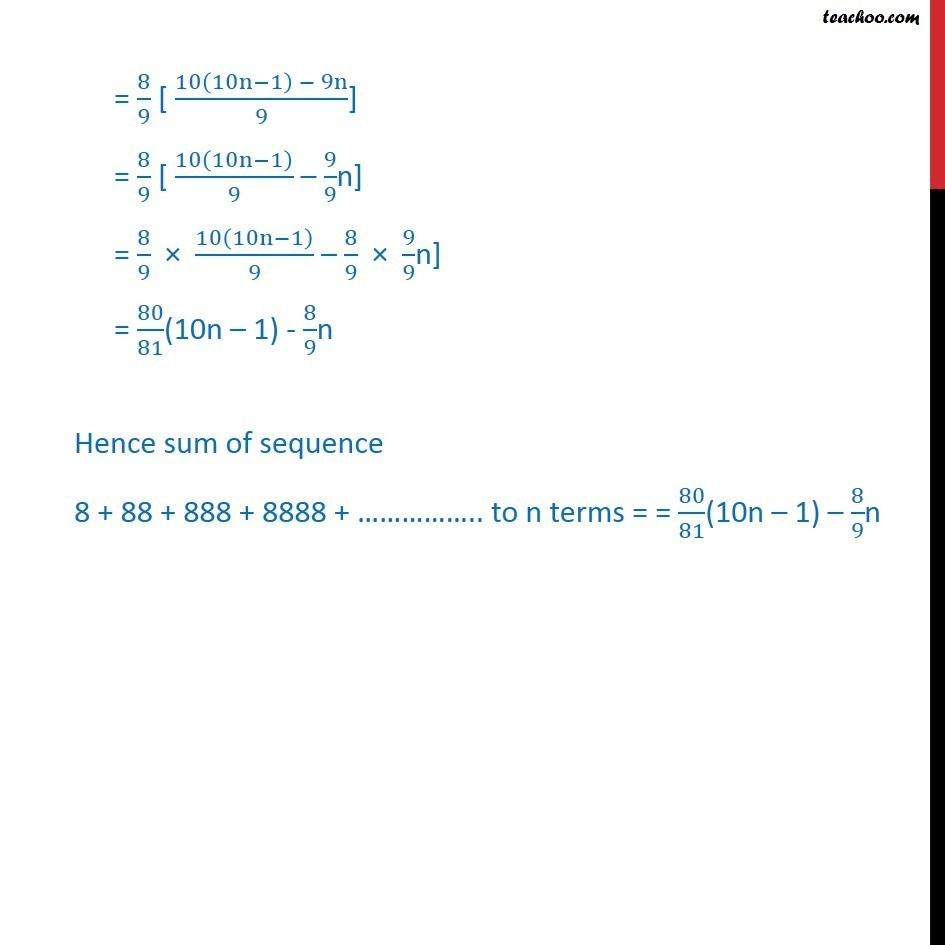Geometric Progression(GP): Formulae based

Chapter 8 Class 11 Sequences and Series
Concept wiseLearn in your speed, with individual attention - Teachoo Maths 1-on-1 Class

### Transcript

Ex9.3, 18 Find the sum to n terms of the sequence, 8, 88, 888, 8888 8, 88, 888, 8888 to n term This is not a GP but we can relate it to a GP By writing as Sum = 8 + 88 + 888 + 8888 + upto n terms = 8(1) + 8(11) + 8(111) + upto n term Taking 8 common = 8(1 + 11 + 111 + upto n term) Divide & multiply by 9 = 8/9[9(1 + 11 + 111 + upto n term)] = 8/9 [ 9 + 99 + 999 + 9999 + upto n terms] Sum = 8/9 [ 9 + 99 + 999 + 9999 + upto n terms] = 8/9 [ (10 1)+(100 1)+(1000 1)+ upto n terms] = 8/9 [ (10 1)+(102 1)+(103 1)+ upto n terms] = 8/9 [ (10 + 102 + 103 upto n terms) (1 + 1 + 1 + upto n terms)] = 8/9 [(10 + 102 + 103 upto n terms) n 1] We will solve (10 + 102 + 103 upto n terms) separately We can observe that this is GP With first term a = 10 & common ratio r = 102/10 = 10 We know that sum of n terms = (a( ^ 1))/( 1) i.e. Sn =(a( ^ 1))/( 1) putting value of a & r Sn = (10(10n 1))/(10 1) Substituting 10 + 102 + 103 + upto n times = (10(10n 1))/9 in (1) Sum = 8/9 [(10 + 102 + 103 + upto n terms) n] = 8/9 [ (10(10n 1))/(10 1) n ] = 8/9 [ (10(10n 1))/9 n ] = 8/9 [ (10(10n 1) 9n)/9] = 8/9 [ (10(10n 1) 9n)/9] = 8/9 [ 10(10n 1)/9 9/9n] = 8/9 10(10n 1)/9 8/9 9/9n] = 80/81(10n 1) - 8/9n Hence sum of sequence 8 + 88 + 888 + 8888 + .. to n terms = = 80/81(10n 1) 8/9n